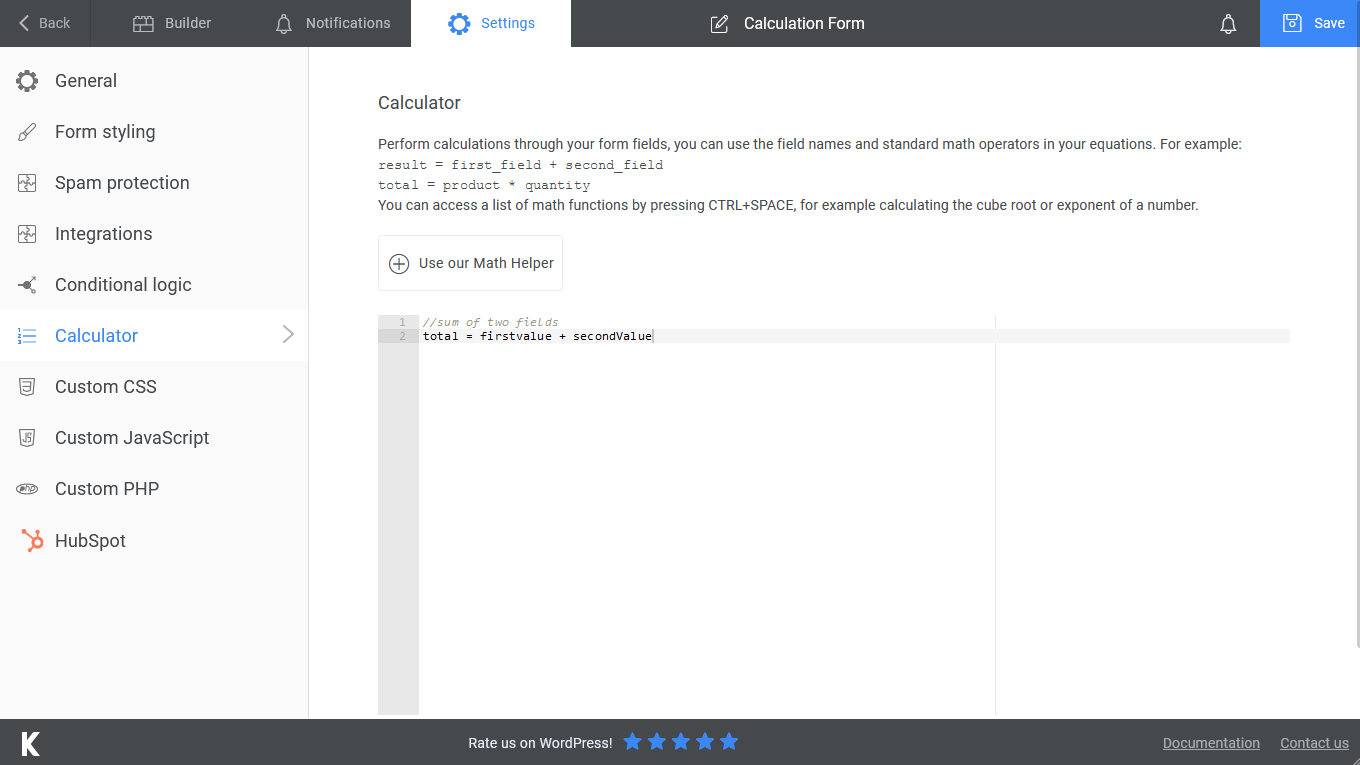1. Home
2. Docs
3. Getting Started
4. Form Calculator

# Form Calculator

You can start performing calculations through your form fields with this easy to use, yet powerful calculator.

You can use different field types for your calculations and also setup chain calculations, for example the sum of the fields can be used as a variable for your next calculation.

First we will need to add the fields that be will part of our calculations. In our first example we will use general operators like adding, subtracting, multiplying and dividing.Let see how we can build our first calculation with Kali Forms.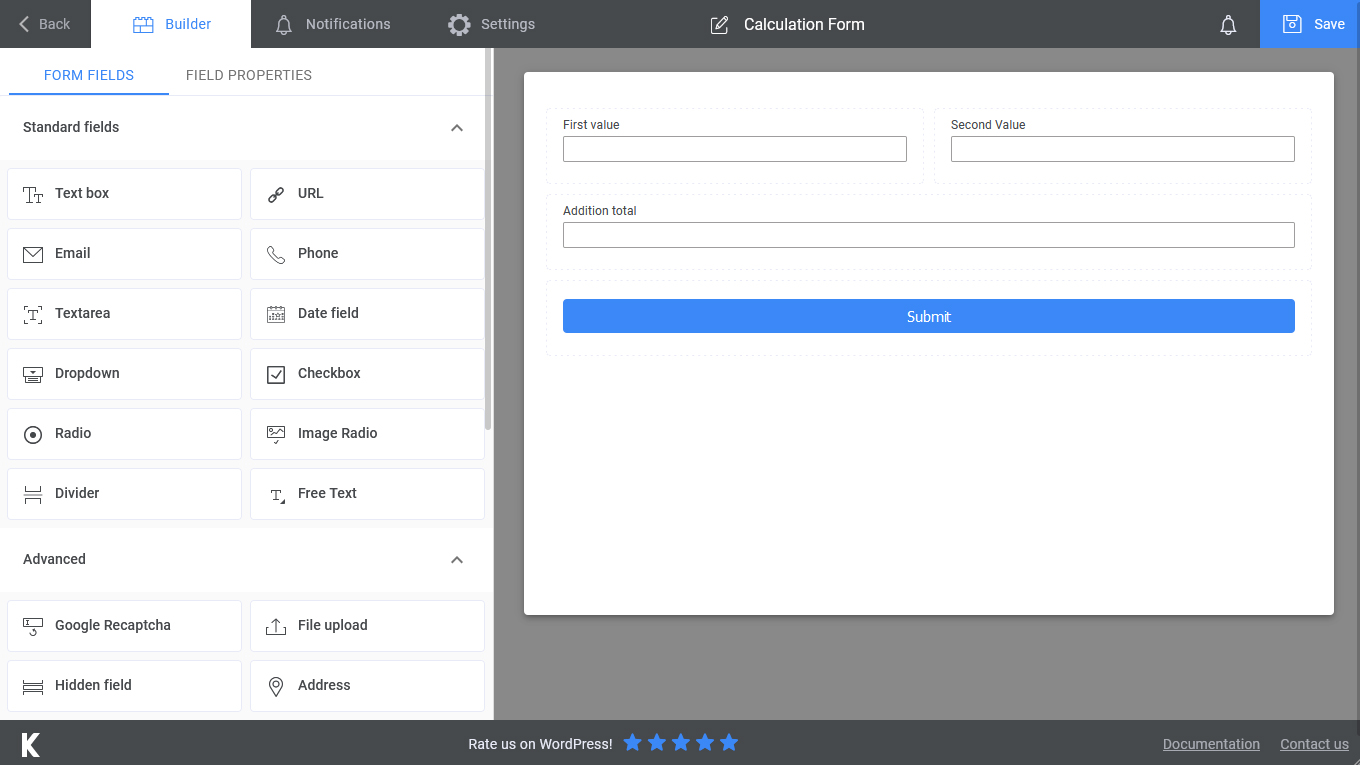After the fields are added we will need to start defining our calculation equations. This can be done by navigating to the Calculator tab in your form configuration.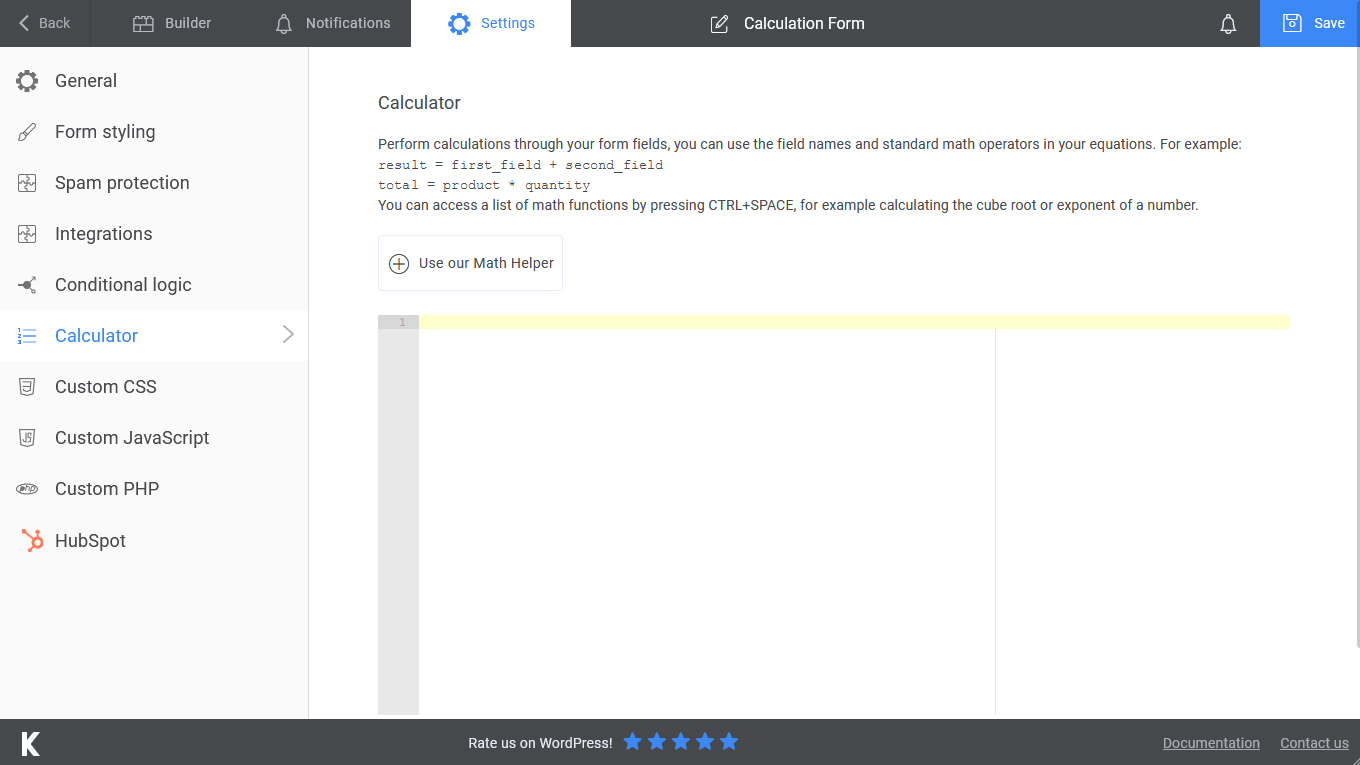• ##### 1. With the help of our Math helper

This will open a dialog box at the bottom of the screen, all you need to do is:

• Set the calculation type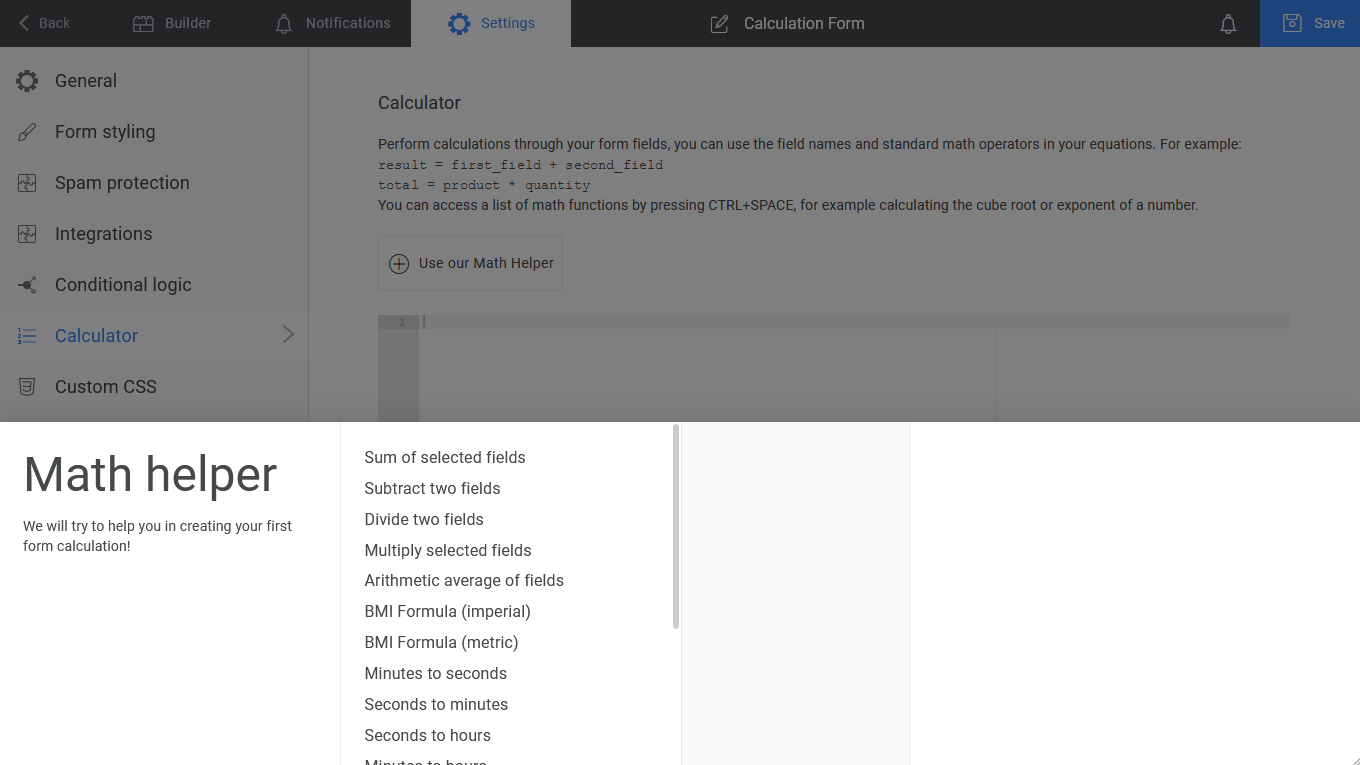• Select the total field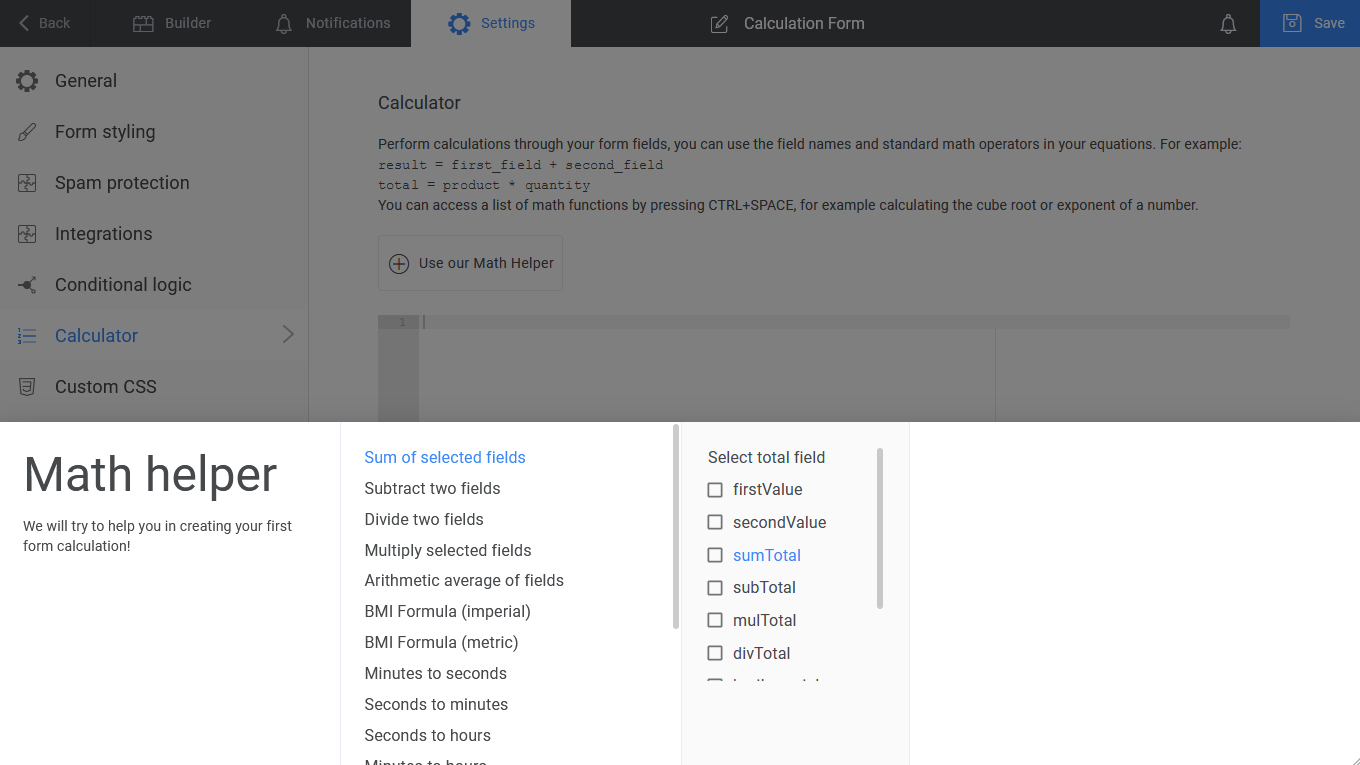• Choose the fields that will be used in the calculation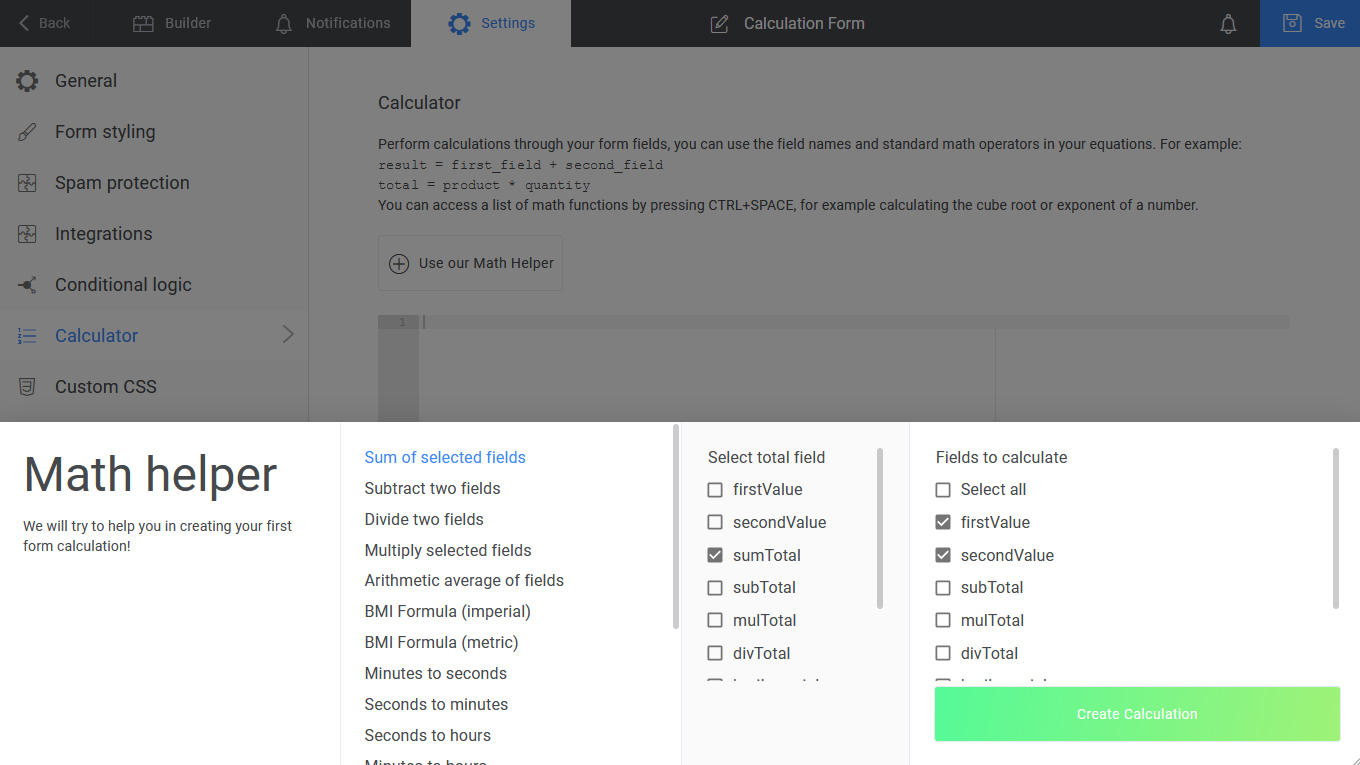When using the Math helper feature you have several functions available that will allow you to perform operations.

Name Operation performed
Subtract two fields Subtracts the second field from the first field.
Divide two fields Divides the first field by the second field.
Multiply selected fields Multiplies the fields you select.
Arithmetic average of fields The sum of all of the numbers in a list divided by the number of items in that list.
BMI Formula(metric) Divide your weight (m) by your height (kg), divide the result by your height again.
Minutes to seconds Converts minutes to seconds.
Seconds to minutes Converts seconds to minutes.
Seconds to hours Converts seconds to hours.
Minutes to hours Converts minutes to hours.
Hour:Minute:Seconds to seconds Convers the time with the format mentioned above to seconds.
Speed Calculates the speed by dividing the distance with the time it took to complete it.
Running pace This divides the total time by the distance you ran.
Running time Calculates the total running time by multiplying your total distance with your pace.
Running distance Calculates the distance by dividing the total time by your pace.
Day difference between dates Calculate the number of days between your chosen dates.
• ##### 2. By directly writing the ecuation in the designated calculations area